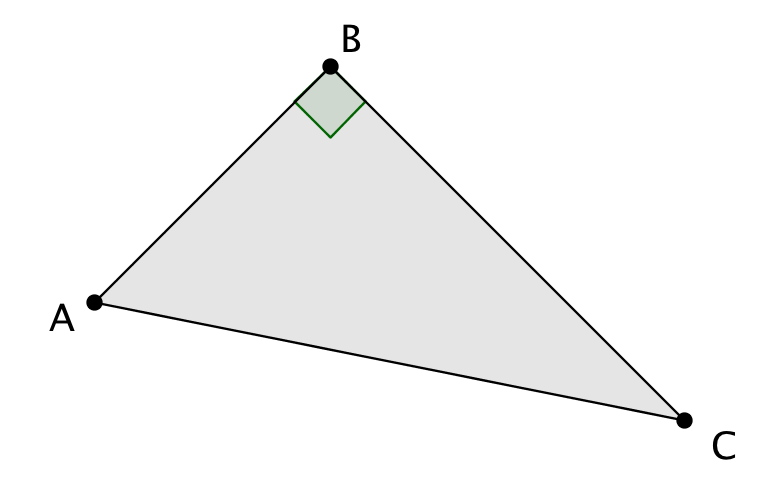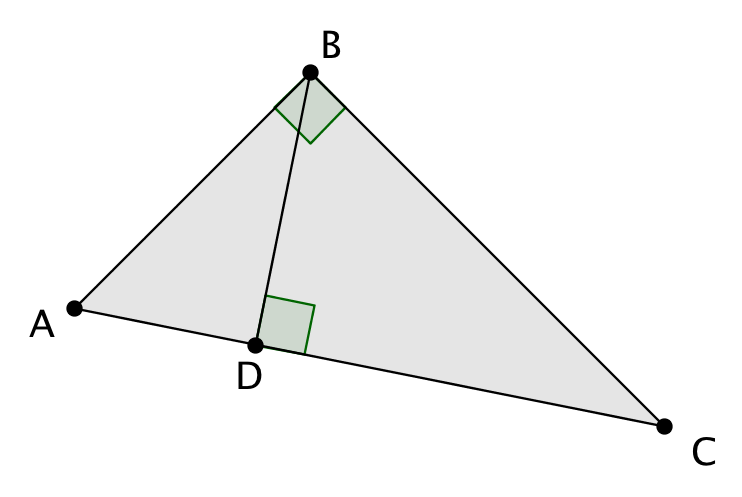# Right Triangles and Trigonometry

## Objective

Define the parts of a right triangle and describe the properties of an altitude of a right triangle.

## Common Core Standards

### Core Standards

?

• G.CO.A.1 — Know precise definitions of angle, circle, perpendicular line, parallel line, and line segment, based on the undefined notions of point, line, distance along a line, and distance around a circular arc.

• G.SRT.B.4 — Prove theorems about triangles. Theorems include: a line parallel to one side of a triangle divides the other two proportionally, and conversely; the Pythagorean Theorem proved using triangle similarity.

?

• 8.G.A.4

• 8.G.B.6

## Criteria for Success

?

1. Describe the parts of a triangle based on their relative position (e.g., adjacent, opposite). Understand that these descriptions apply to right and non-right triangles.
2. Describe the right triangle–specific relationships of hypotenuse (side opposite the right angle) and legs (sides adjacent to each other and the right angle).
3. Recall altitudes of triangles as line segments that connect the vertex of a triangle with the opposite side and intersect the opposite side in a right angle.
4. Explain how you know that when a triangle is divided using an altitude, the two triangles formed are similar.

## Tips for Teachers

?

This lesson, specifically Criteria for Success 3, connects to Unit 2, Lesson 11 because the altitude of an isosceles triangle is the perpendicular bisector.

## Anchor Problems

?

### Problem 1

Annotate the following diagram with the vocabulary words of “leg” and “hypotenuse.”### Problem 2

Given: ${\overline{BD}}$ is the altitude of right triangle ${\triangle ABC}$ through right angle ${\angle B}$
Prove: ${\triangle ABD\sim \triangle BCD}$## Problem Set

?

The following resources include problems and activities aligned to the objective of the lesson that can be used to create your own problem set.

• Include problems where students create proportions using side lengths to determine the relationship between the sides of the triangles.

Find the measure of ${AD}$ and ${DB}$ given:
${AC=16}$
${AB=8}$
${BC=12}$